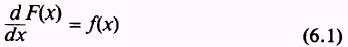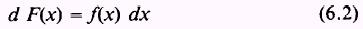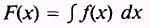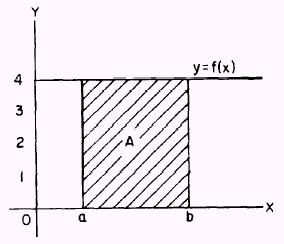calculus. Basically, integration is the inverse of differentiation just as division is the inverse of multiplication, and as subtraction is the inverse of addition."> Chapter 6 - IntegrationCustom SearchCHAPTER 6 INTEGRATION LEARNING OBJECTIVES Upon completion of this chapter, you should be able to do the following: 1. Define integration. 2. Find the area under a curve and interpret indefinite integrals. 3. Apply rules of integration. 4. Apply definite integrals to problem solving. INTRODUCTION The two main branches of calculus are differential calculus and integral calculus. Having studied differential calculus in previous chapters, we now turn our attention to integral calculus. Basically, integration is the inverse of differentiation just as division is the inverse of multiplication, and as subtraction is the inverse of addition. DEFINITIONS Integration is defined as the inverse of differentiation. When we were dealing with differentiation, we were given a function F(x) and were required to find the derivative of this function. In integration we will be given the derivative of a function and will be required to find the function. That is, when we are given the function f(x), we will find another function, F(x), such thatIn other words, when we have the function f(x), we must find the function F(x) whose derivative is the function f(x). If we change equation (6.1) to readwe have used dx as a differential. An equivalent statement for equation (6.2) isWe call f(x) the integrand, and we say F(x) is equal to the indefinite integral of f(x). The elongated S,, is the integral sign. This symbol is used because integration may be shown to be the limit of a sum. INTERPRETATION OF AN INTEGRAL We will use the area under a curve for the interpretation of an integral. You should realize, however, that an integral may represent many things, and it may be real or abstract. It may represent the plane area, volume, or surface area of some figure.Figure 6-1.-Area of a rectangle.Integrated Publishing, Inc. - A (SDVOSB) Service Disabled Veteran Owned Small Business